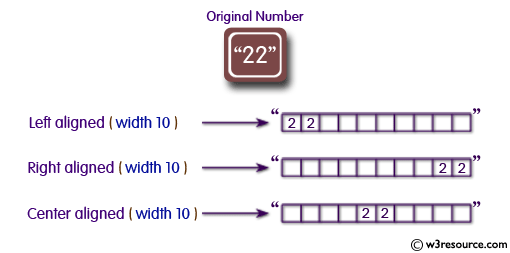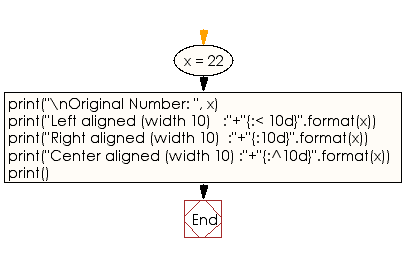﻿ Python: Display a number in left, right and center aligned of width 10 - w3resource# Python: Display a number in left, right and center aligned of width 10

## Python String: Exercise-37 with Solution

Write a Python program to display a number in left, right and center aligned of width 10.Sample Solution:-

Python Code:

``````x = 22
print("\nOriginal Number: ", x)
print("Left aligned (width 10)   :"+"{:< 10d}".format(x));
print("Right aligned (width 10)  :"+"{:10d}".format(x));
print("Center aligned (width 10) :"+"{:^10d}".format(x));
print()
```
```

Sample Output:

```Original Number:  22
Left aligned (width 10)   :22
Right aligned (width 10)  :        22
Center aligned (width 10) :    22
```

Flowchart:## Visualize Python code execution:

The following tool visualize what the computer is doing step-by-step as it executes the said program:

Python Code Editor:

Have another way to solve this solution? Contribute your code (and comments) through Disqus.

What is the difficulty level of this exercise?

Test your Programming skills with w3resource's quiz.

﻿

## Python: Tips of the Day

What is the difference between Python's list methods append and extend?

append: Appends object at the end.

```x = [1, 2, 3]
x.append([4, 5])
print (x)
```

Output:

```[1, 2, 3, [4, 5]]
```

extend: Extends list by appending elements from the iterable.

```x = [1, 2, 3]
x.extend([4, 5])
print (x)
```

Output:

```[1, 2, 3, 4, 5]
```

Ref: https://bit.ly/2AZ6ZFq